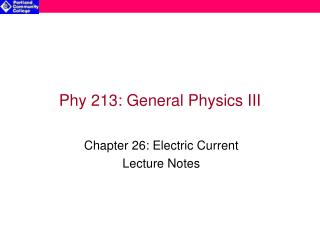DownloadDownload PresentationPhy 213: General Physics III

# Phy 213: General Physics III

Télécharger la présentation## Phy 213: General Physics III

- - - - - - - - - - - - - - - - - - - - - - - - - - - E N D - - - - - - - - - - - - - - - - - - - - - - - - - - -
##### Presentation Transcript

1. Phy 213: General Physics III Chapter 26: Electric Current Lecture Notes

2. Electric Current • An electric potential causes electric charges to move • The flow of electric charge is called electric current • Positive charge accelerates toward lower electric potential • Negative charge accelerates toward higher electric potential • The rate of flow of electric charge (i) through a conducting material is • The SI units are coulombs per second (C/s), called amperes (A): 1 C/s = 1 A • The quantity of charge movement (q) is related to the electric current (i): Note:By convention, electric current is defined as the flow of positive charge carriers flowing from high potential (+) to low potential (-)

3. Conduction depends on potential difference between 2 regions & how far apart those regions are separated Increasing the cross-sectional area increases amount of charge that can flow through in a given time, for constant J i VA VA VB L L i VB A Conduction Model of Electric Current(through a constant area pathway) • Of course, the relative ability to conduct charge is an intrinsic property of different materials !!

4. Conduction of Electric Current (cont.) • The rate of charge flow (current) w/in a conductor depends on • The potential difference between 2 regions along the conducting pathway material (DV) • The cross sectional area of the conducting pathway (A) • The ability of the conductor to conduct charge (s) {the conductivity} • The length of conducting pathway (L) • For a uniform cylindrical conductor, combining these elements leads to the conduction equation: The quantity, , is called the conductivity (g): • Conductivity is more commonly expressed as the “resistance” (R) of the conducting pathway: where r is the “resistivity “ (in units of or )

5. L A Current Density & Drift Velocity • For a steady state uniformly distributed electric current, the current can be expressed in terms of its associated current density vector (J). where is the area vector, perpendicular to the cross sectional area through which the current flows • The current density of charge flow through a cylindrical region (area, A and length L) is related to the number of charge carriers (n) & their average drift velocity (vdrift): For a 0.1A current flowing through a copper wire (n = 8.49x1028m-3 & A = 1x10-6 m2):

6. Ohm’s Law • The electric current can be re-expressed as: where DV = V = the potential difference across the conductive pathway • Thus, the current through any conducting pathway can be described by dividing the potential across the pathway by the effective resistance of the pathway… Or The resistance of a conducting pathway is defined as the potential difference divided by the current flow: • When the ratio, V/i, is a constant value for all values of V the conductor is said to be an “Ohmic” material: V/i = R = constant {Ohm’s Law} • When R is not constant, the conductor is non-Ohmic • Ohm’s Law is more commonly expressed as: V = iR

7. Resistance • The resistance (R) of a substance depends on: • length (L) • Cross-sectional area (A) • Resistivity (r)  units are W.m • Units of resistance are V/A, called ohms (W) 1 V/A = 1 W • The intrinsic physical property, resistivity (r) depends on temperature. Over a limited temperature range the temperature dependence is given by: • For conductors: resistivity increases with T (a > 0) • For semi-conductors: resistivity decreases with T (a < 0)

8. Table of Selected Resistivities (@ 20oC) Table reproduced from: http://hyperphysics.phy-astr.gsu.edu

9. Relation between Current Density & Resistance The resistivity of a conducting material, reflects the ability of the material to resist current flow and thus limit current density, for a given potential difference: where n = V/L (the potential difference per unit length) Semi-Obvious Consequence: For steady state current flow, as the current density increases and surface area decreases: r=constant→V/L must increase→ V will increase →R must increase

10. Power • It requires energy to “push” charge through an electrical device. This should be obvious given that the charge flows from high potential (Uhigh) to lower potential (Ulow). • The energy must go somewhere if the charge flows at constant drift velocity (DK=0). It is radiated out as heat energy. • The energy transfer associated with moving a charge dq across a potential difference, V is: • The rate of energy transfer, in Watts, is given by: • Applying Ohm’s Law, the power can be alternatively expressed to describe “resistive” dissipation of energy: